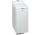Perimeter - 7th grade (12y) - examples

1. Triangular prismCalculate a triangular prism if it has a rectangular triangle base with a = 4cm and hypotenuse c = 50mm and height of the prism is 0.12 dm.
2. GardenArea of a square garden is 6/4 of triangle garden with sides 56 m, 35 m, and 35 m. How many meters of fencing need to fence a square garden?
3. WasherWashing machine drum wash at 55 RPM. Washing machine motor pulley has diameter 6 cm. What must be the diameter of the drum machine pulley when the motor is at 333 RPM?
4. Right triangle AlefThe obvod of a right triangle is 84 cm, the hypotenuse is 37 cm long. Determine the lengths of the legs.
5. CylindersArea of the side of two cylinders is same rectangle of 50 cm × 11 cm. Which cylinder has a larger volume and by how much?
6. Circle arcCircle segment has a circumference of 135.26 dm and 2096.58 dm2 area. Calculate the radius of the circle and size of central angle.
7. Two squaresTwo squares whose sides are in the ratio 5:2 have sum of its perimeters 73 cm. Calculate the sum of area this two squares.
8. ConeCircular cone of height 15 cm and volume 5699 cm3 is at one-third of the height (measured from the bottom) cut by a plane parallel to the base. Calculate the radius and circumference of the circular cut.
9. Earth parallelEarth's radius is 6370 km long. Calculate the length parallel of latitude 50°.
10. ArcCircle arc corresponding to angle is 32° is 28 dm long. What is the length of the entire circle?
11. Mr. ZucchiniMr. Zucchini had a rectangular garden whose perimeter is 28 meters. Content area of the garden filled just four square beds, whose dimensions in meters are expressed in whole numbers. Determine what size could have a garden. Find all the possibilities and
12. Quarter circularThe wire that is hooked around the perimeter of quarter-circular arc has length 3π+12. Determine the radius of circle arc.
13. CapJesters hat is shaped a rotating cone. Calculate how much paper is needed to the cap 60 cm high when head circumference is 52 cm.
14. RhombusFind the length of the other diagonal and area of rhombus. The perimeter of a rhombus is 40 cm and one of the diagonals is of length 10 cm.
15. PrismThree cubes are glued into prism, sum of the lengths of all its edges is 115 cm. What is the length of one edge of the original cube?
16. Bicycle wheelA bicycle wheel has a diameter of 60 cm. Approximately how many times does wheel rotate at 2.5 km long trip?
17. CircleHow big is area of circle if its circumference is 80.6 cm?Determine the radius of the circle, if its perimeter and area is the same number.Equilateral triangle with side 40 cm has the same perimeter as an isosceles triangle with arm of 45 cm. Calculate the base x of an isosceles triangle.How long path will walk the end of the clock's minute-hand long 7 cm for 330 minutes? How long will walk in the same time hour-hand?